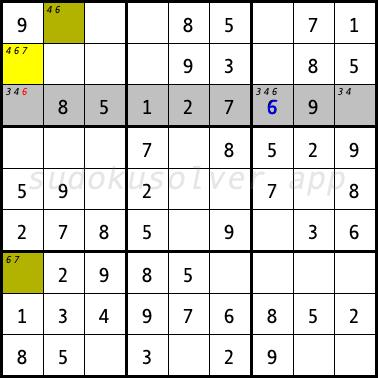# XYZ-Wing

The XYZ-Wing technique is an extension of the XY-Wing technique. The pivot also contains the candidate Z. Therefore, either the Z digit is placed in the pivot or in the pincers if the pivot has an X or Y digit. Thus, the Z digit can be eliminated from the cells that see three cells forming the XYZ-Wing simultaneously.

In the sudoku diagram below, the XYZ-Wing is formed by the R2C1 cell (pivot) and the R1C2 and R7C1 cells (pincers, X = 4, Y = 7, Z = 6). If the R2C1 cell is set to 4, then the R1C2 cell will be set to 6. If the R2C1 cell is set to 7, then the R7C1 cell will be set to 6. If R2C1 is not set to 4 or 7, then it can only get the digit 6. So one of the three cells forming the XYZ-Wing will have the digit 6. Hence the digit 6 can be eliminated from the cells that see simultaneously the cells R2C1, R1C2 and R7C1, i.e. from the cell R3C1. Thanks to this elimination, the digit 6 in the third row can only be placed in the R3C7 cell.